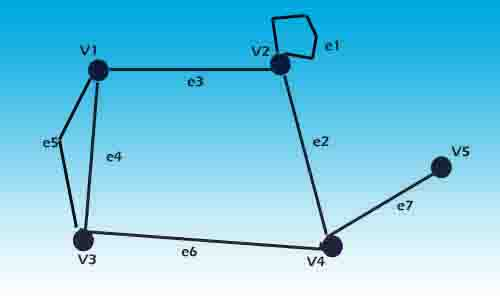# Graph: Types of the graph in data structure 2019? / What is graph terminology in data structure?

A graph(Types of the graph in a data structure) is a structure containing a (V, E) set of objects. Here V represents the vertices or nodes and E represents the edges or arcs. Say V= {v1, v2, v3, v4, v5} and E = {e1, e2, e3, e4}. Each edge Ek is identified with an unordered pair (Vi, Vj) of vertices. The vertices Vi and Vj associated with edge Ek are called the end vertices of Ek. You can see the graph below.Fig1: Graph: Types of the graph in data structure

An edge that has the same vertices as both of its end vertices is called a self-loop. Edge e1 is the self-loop in Fig1 above. And if more than one edge is associated with one set of end vertices then vertices are referred to as parallel edges. Edges e4 and e5 in the above figure are parallel edges.|Types of the graph in data structure|

Within this blog post, we are going to cover What are the types of graphs in the data structure? What is the graph and types of graphs in the data structure? What are a Graph and its types?….

…. What is graph terminology in data structure? What is a graph in a data structure, a Graph in data structure with an example, a Graph and its types in the data structure, a Directed graph in the data structure, or a Simple graph in a data structure?|Types of the graph in data structure|

## Types of the graph in data structure:

On the basis of vertices order and edges, the connections graph is also classified into various categories.

### Undirected Graph:

A graph is called an undirected graph if there is the same edge say Ek identified with a different pair of vertices say (Vi, Vj) and (Vj, Vi).

Let’s understand this with a more simple explanation. When we go from V1 to V2 and V2 to V1 in both cases we get the same edge e1 as the resulting edge.

Set of vertices V= {v1, v2, v3, v4}

Set of edges E = {e1, e2, e3, e4}

In this graph pairs (v1, v2) and (v2, v1) represent the same edge.

### Directed Graph:

In a directed graph, every edge of the graph is identified by an ordered pair of vertices. it is also known as a digraph, so don’t be confused with this other name of a directed graph.

In this graph, there exists some mapping, that maps each edge of a graph onto some ordered pair of vertices(Vi, Vj).

The first element of an ordered pair of vertices is referred to as the start vertex and the second element is known as the end vertex. Fig3 shows the directed graph.

Vertex pair (Vi, Vj) reads as Vi-Vj an edge is directed from Vi to Vj.

### Complete Graph:

A simple graph In which there exists an edge between every pair of vertices is called a complete graph. A complete graph is also known as a universal graph or a clique. Fig 4 shows complete graphs of two, three, four, and five vertices.

### Connected Graph:

A graph G is known as a connected graph if there is at least one path between every pair of vertices in G. Otherwise, G is disconnected. See the below image for a connected graph.

### Multi Graph:

A graph that contains a pair of nodes joined by more than one edge is called a multigraph and such edges are called parallel edges. See the image below for the multigraph.

You can also go through a few more amazing blog links below related to Data Structure:

## Conclusion:

In this above blog post(Types of the graph in the data structure), you have learned about the graph and types of graphs in the data structure. A graph is a shape that is built with the help of vertices or nodes and edges. and on the basis of their property, they are further classified into different types like a simple graph, undirected graph, directed graph, connected graph, complete graph, and multigraph. With a change in the order of vertices and edges connection a graph nature changes and it is known by some other name. A graph is used for solving many mathematical problems and it is used to get an optimal solution for a problem.

So, inside this blog post(Types of the graph in a data structure), we have learned various types of graph-like What are the types of graphs in data structure? What is graph and types of graphs in the data structure? What are a Graph and its types? What is graph terminology in data structure?…..

…What is a graph in the data structure, Graph in data structure with an example, Graph and its types in the data structure, Directed graph in the data structure, Simple graph in the data structure

In the case of any queries, you can write to us at a5theorys@gmail.com we will get back to you ASAP.

Hope! you would have enjoyed this post(Types of the graph in the data structure) about the graph in the data structure and the types of graphs or classification of a graph.

Please feel free to give your important feedback in the comment section below.|Types of the graph in data structure|

Have a great time! Sayonara!#### Anurag

I am a blogger by passion, a software engineer by profession, a singer by consideration and rest of things that I do is for my destination.